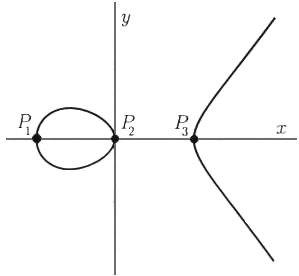A# elliptic curveAn elliptic curve is the set of solutions to a type of cubic equation whose solutions lie on a torus (a donut-shaped surface). The particular type of cubic equation whose solutions lead to elliptic curves takes the form

y2 + axy + by = x3 + cx2 + dx + e.

Elliptic curves, which are said to have a genus of 1, have an unusually rich theory and structure, and their study is linked to many other important areas of mathematics and their applications. For example, it was work done on elliptic curves by Andrew Wiles that finally led to a proof of Fermat's last theorem.